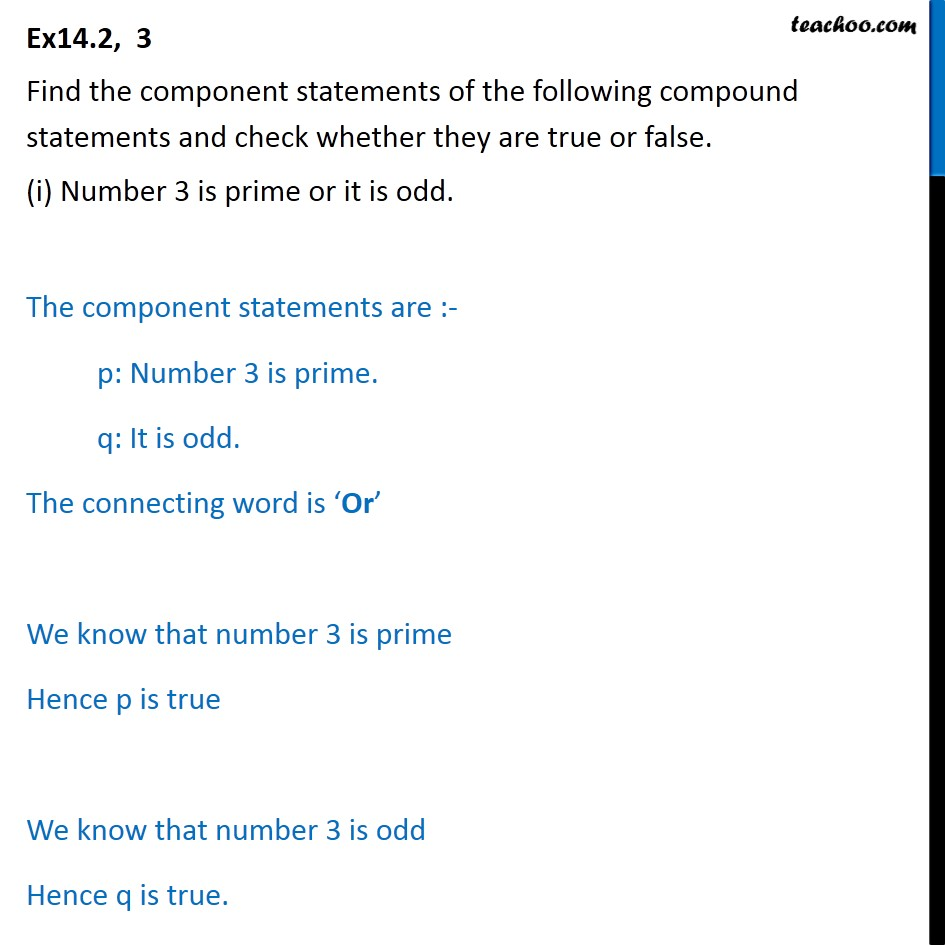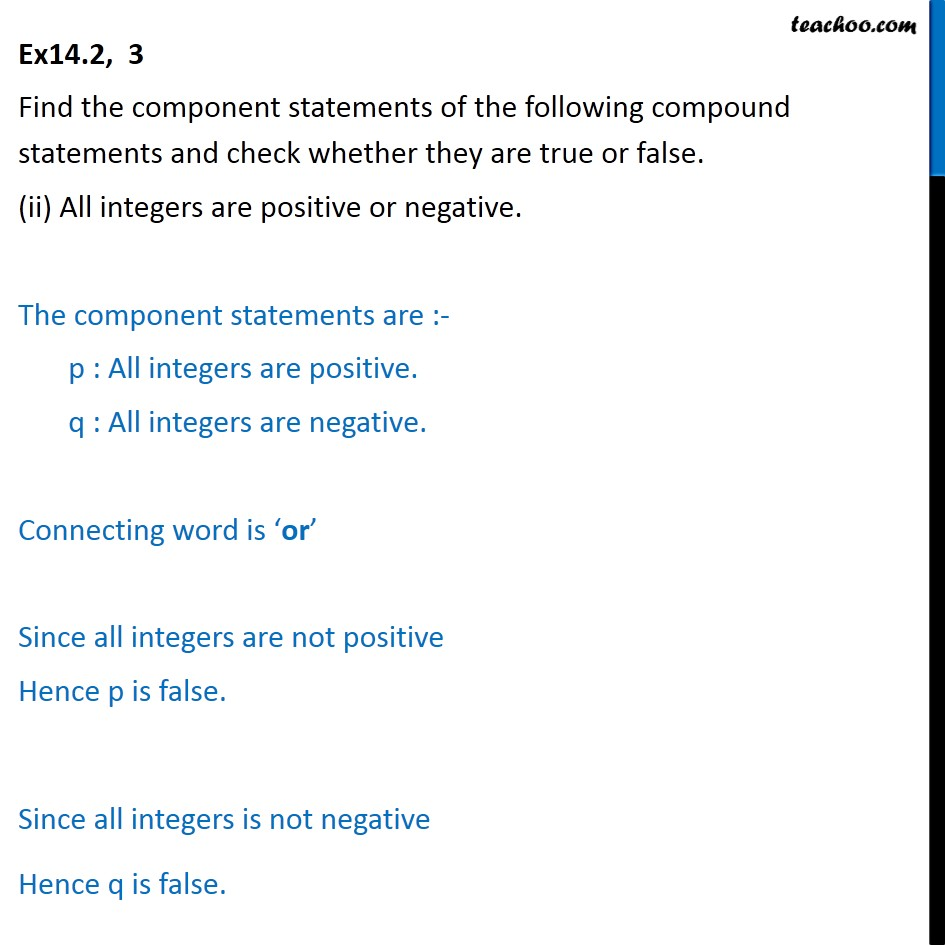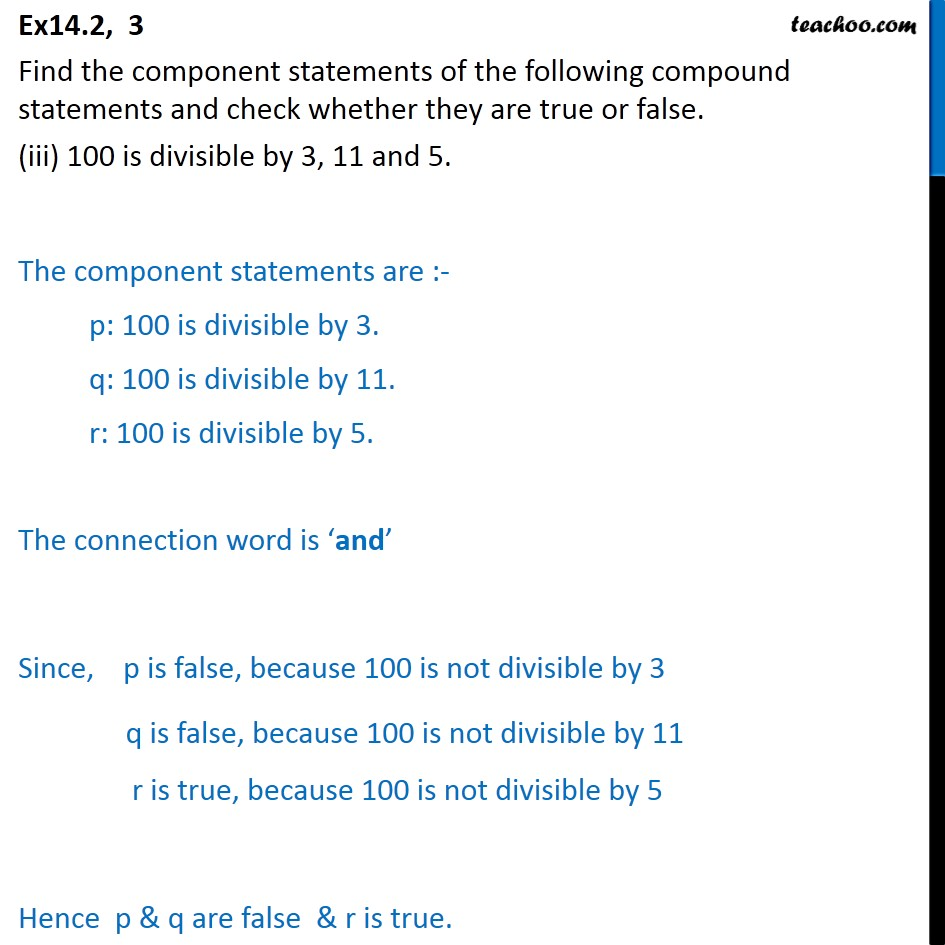1. Chapter 14 Class 11 Mathematical Reasoning
2. Concept wise
3. Words 'And' & 'Or'

Transcript

Ex 14.2, 3 Find the component statements of the following compound statements and check whether they are true or false. (i) Number 3 is prime or it is odd. The component statements are :- p: Number 3 is prime. q: It is odd. The connecting word is ‘Or’ We know that number 3 is prime Hence p is true We know that number 3 is odd Hence q is true. Ex14.2, 3 Find the component statements of the following compound statements and check whether they are true or false. (ii) All integers are positive or negative. The component statements are :- p : All integers are positive. q : All integers are negative. Connecting word is ‘or’ Since all integers are not positive Hence p is false. Since all integers is not negative Hence q is false. Ex14.2, 3 Find the component statements of the following compound statements and check whether they are true or false. (iii) 100 is divisible by 3, 11 and 5. The component statements are :- p: 100 is divisible by 3. q: 100 is divisible by 11. r: 100 is divisible by 5. The connection word is ‘and’ Since, p is false, because 100 is not divisible by 3 q is false, because 100 is not divisible by 11 r is true, because 100 is not divisible by 5 Hence p & q are false & r is true.

Words 'And' & 'Or'